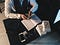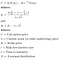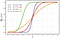Image by Kuncheek from Pexels

Part 2: Nvidia CUDA tutorial (with code) - how to use GPU computing power to boost speed of options pricing valuation. Black-Scholes-Merton model boosted by CUDA in c++.

Note: Part 1 may be found here — where I run tests of Python vs C++ vs CUDA performance.

There are millions of financial transactions each day globally. The vast majority is conducted on a market for derivatives (options, futures etc., are typical examples). This means that, every day, thousands of financial institutions (like: banks, stock exchanges, etc.) have to value its financial holdings.

Note: here is the link to the latest very interesting case connected with the pricing of derivatives.

In this tutorial, we’re going to introduce CUDA as a solution to speed up calculations for evaluation of options.

What is an option, and what’s the formula (in very short)?

Simply, an option is a financial contract. A Buyer has an option (can decide) to buy/sell an underlying security. Actually, underlying security may mean anything, but usually it’s currency, stock or bond. Very simply, it means that the buyer can decide whether to buy/sell the asset at the current price on the market.

The formula for the valuation of options is a little complicated at the first glance. In fact the options' formula, known as the holy grail of investing, caused many banks to crash. It seems that rational models do not work properly in a greed and irrational human environment.

Here is the formula:Taken from Wikipedia

As you can see, the above formula takes as parameters current price, strike price, time and interest rate. Options are priced based on a normal distribution assumption (which in reality may not hold).

OK, let’s go to the code!

Fortunately for us, CUDA devs prepared implementation for pricing options here. I’m going to use this code, explain key details and run it on my PC.

There are three files:

• BlackScholes.cu
• BlackScholes_gold.cpp
• BlackScholes_kernel.cuh

# BlackScholes.cu file

The file consists of the main function, that is responsible for executing the whole program for pricing of options.

The key parts here are:

1. Find cuda device helper — it is stored in a helper_functions.h. This function simply checks whether there is an NVIDIA GPU available in the machine.
`findCudaDevice(argc, (const char **)argv);`

2. Malloc function — allocates a block of memory and returns a void pointer to the first byte of the allocated memory block. In our case we need to allocate memory of each data we are going to use.

`h_CallResultCPU = (float *)malloc(OPT_SZ);`

And we also need to free memory in the way:

`free(h_CallResultCPU);`

3. Malloc in cuda — allocates a block of memory in a GPU. Next, we’re going to copy data from a host to GPU.

`checkCudaErrors(cudaMalloc((void **)&d_CallResult, OPT_SZ));`

After calculation, we need to free a memory using:

`checkCudaErrors(cudaFree(d_CallResult));`

4. Memory copy cuda — it copies data from host to GPU so we are able to use GPU and make calculation.

`checkCudaErrors(cudaMemcpy(d_StockPrice, h_StockPrice, OPT_SZ, cudaMemcpyHostToDevice));`

5. CUDA’s special`<<<1, 1>>>`syntax. It tells a GPU device to perform a given operation defined by __global__ function. The first parameter stands for a number of blocks. The second parameter is for a number of threads in a thread block. Here is a detailed explanation for this:

`BlackScholesGPU<<<DIV_UP((OPT_N/2), 128), 128>>>`

In our case DIV_UP is used to dynamically decide on a number of blocks. Let’s see an example numbers like:

`#include <iostream>using namespace std;#define DIV_UP(a, b) ( ((a) + (b) — 1) / (b) )int main(){ cout<<DIV_UP(128, 128) << endl; cout<<DIV_UP(400, 128) << endl; cout<<DIV_UP(1000, 128) << endl;  return 0;}`

code outputs:

`148`

Note: for quick tests you can use an online c++ compiler here.

# File BlackScholes_gold.cpp

The file consists of c++ implementation of black-scholes-merton model. The code is run on a CPU to serve as a benchmark and validator of results given by GPU.

The code is pretty straight forward, there are only three functions with below parameters:

`static double CND(double d)static void BlackScholesBodyCPU(float &callResult,float &putResult,float Sf, //Stock pricefloat Xf, //Option strikefloat Tf, //Option yearsfloat Rf, //Riskless ratefloat Vf  //Volatility rate)extern "C" void BlackScholesCPU(float *h_CallResult,float *h_PutResult,float *h_StockPrice,float *h_OptionStrike,float *h_OptionYears,float Riskfree,float Volatility,int optN)`

Function CND: This function approximates a cumulative distribution function:Taken from wikipedia

Function BlackScholesBodyCPU: This function calculates call and put price of an option.

Function BlackScholesCPU: This function is actually a loop to calculate many options with different parameters for testing.

# File BlackScholes_kernel.cuh

The file consists of the same c++ code as in above .cpp file with slight additional syntax code.

1. __global__ function is also called “kernel” function. It’s the function that you may call from the host side using CUDA kernel call semantics (`<<<...>>>`). In our case it’s defined in the above file a BlackScholesGPU function.
`__global__ void BlackScholesGPU`

2. __launch_bounds__ function is used to specify manually number of registers for a program. What are registers then? — registers are very fast computer memory which are used to execute programs and operations efficiently.

`__launch_bounds__(128)`

3. __device__ function can be called only from the device (GPU), and it is executed only in the device. This is very similar to __global__, but can be called only from a device.

`__device__ inline void BlackScholesBodyGPU`

Let’s run the program and see output:

Note: program is executed on Windows 10 and NVIDIA RTX2080 Super (without boost enabled).

As for starting point I left original data configured by CUDA devs:

`const int OPT_N = 4000000;const int NUM_ITERATIONS = 512;const int OPT_SZ = OPT_N * sizeof(float);const float RISKFREE = 0.02f;const float VOLATILITY = 0.30f;`

And here is the final output:

`GPU Device 0: “Turing” with compute capability 7.5Initializing data……allocating CPU memory for options.…allocating GPU memory for options.…generating input data in CPU mem.…copying input data to GPU mem.Data init done.Executing Black-Scholes GPU kernel (512 iterations)…Options count : 8000000BlackScholesGPU() time : 2.458114 msec Effective memory bandwidth: 32.545271 GB/sGigaoptions per second : 3.254527BlackScholes, Throughput = 3.2545 GOptions/s, Time = 0.00246 s, Size = 8000000 options, NumDevsUsed = 1, Workgroup = 128Reading back GPU results…Checking the results……running CPU calculations.Comparing the results…L1 norm: 1.787766E-07Max absolute error: 1.192093E-05Shutting down……releasing GPU memory.…releasing CPU memory.Shutdown done.[BlackScholes] — Test SummaryNOTE: The CUDA Samples are not meant for performance measurements. Results may vary when GPU Boost is enabled.Test passed`

For 4 000 000 (actually 8kk) options it took 2.458114 msec (0.00246 s) — Amazing!

All the best!